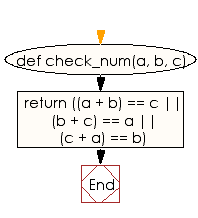﻿ Ruby Basic exercises: Check three given integers and return true if it is possible to add two of the integers to get the third - w3resource# Ruby Basic Exercises: Check three given integers and return true if it is possible to add two of the integers to get the third

## Ruby Basic: Exercise-47 with Solution

Write a Ruby program to check three given integers and return true if it is possible to add two of the integers to get the third.

Ruby Code:

``````def check_num(a, b, c)
return ((a + b) == c || (b + c) == a || (c + a) == b)
end

print check_num(9, 12, 21),"\n"
print check_num(0, -5, -5),"\n"
print check_num(-5, 7, 2),"\n"
print check_num(6, 5, 4)
``````

Output:

```true
true
true
false
```

Flowchart:Ruby Code Editor: